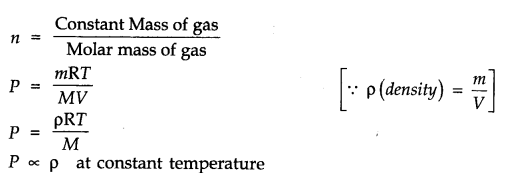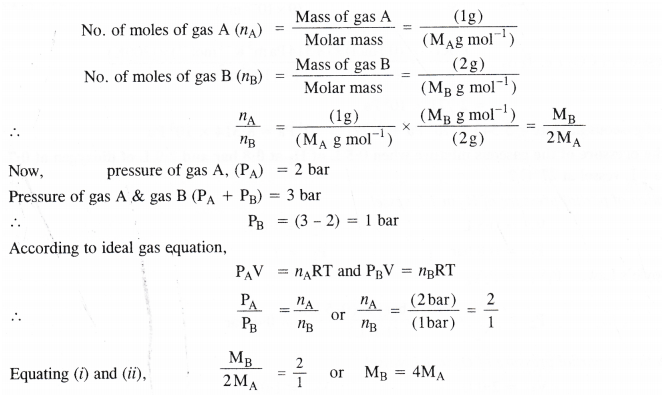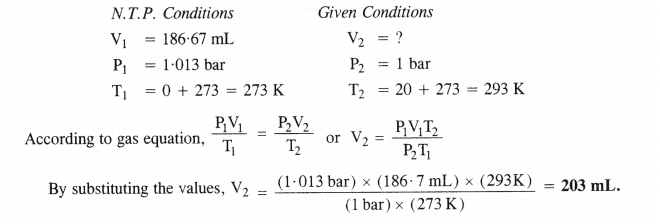• +91 9971497814
• info@interviewmaterial.com

# Chapter 5- States of Matter Interview Questions Answers

### Related Subjects

Question 1 : What will be the minimum pressure required to compress 500 dm3 of air at 1 bar to 200 dm3 at 30°C?

P1 = 1 bar,P2 = ?      V1= 500 dm3 ,V2=200 dm3
As temperature remains constant at 30°C,
P1V1=P2V2
1 bar x 500 dm3 = P2 x 200 dm3 or P2=500/200 bar=2.5 bar

Question 2 : Avessel of 120 mL capacity contains a certain amount of gas at 35°C and 1.2 barpressure. The gas is transferred to another vessel of volume 180 mL at 35°C.What would be its pressure?

V1= 120 mL, P1=1.2 bar,
V2 = 180 mL, P2 = ?
As temperature remains constant, P1V1 =P2V2
(1.2 bar) (120 mL) = P2 (180mL)

Question 3 : Using the equation of state pV=nRT; show that at a given temperature density of a gas is proportional to gas pressure p.

Answer 3 : According to ideal gas equation
PV = nRT or PV=nRT/VQuestion 4 : At 0°C, the density of a gaseous oxide at 2 bar is same as that of dinitrogen at 5 bar. What is the molecular mass of the oxide?

Usingthe expression, d =MP/RT , at the same temperature and for same density,
M1P1 = M2P2 (as R is constant)
(Gaseous oxide) (N2)
or
M1 x 2 = 28 x5(Molecular mass of N2 =28 u)
or M1 = 70u

Question 5 : Pressure of lg of an ideal gas A at 27°C is found tobe 2 bar. When 2g of another ideal gas B is introduced in the same flask atsame temperature, the pressure becomes 3 bar. Find the relationship betweentheir molecular masses.

Answer 5 : Let MA and MB be the molar masses of the two gases Aand B. According to available data :Question 6 : The drain cleaner, ‘Drainex’ contains small bits ofaluminium which react with caustic soda to produce hydrogen. What volume ofhydrogen at 20°C and one bar will be released when 0.15 g of aluminium reacts ?

Answer 6 : Step I. Calculation of thevolume of hydrogen released under N. T.P. conditions.
The chemical equation for the reaction is :
2Al + 2NaOH + 2H2O →2NaAl02 + 3H2
2×27=54 g Sod. meta aluminate 3×22400 mL
54g of Al at N.T.P. release H2 gas= 3 × 22400 mL
0.15g of Al at N.T.P. release H2 gas=
(3×22400mL)×(0.15g)(54g) =186.7mL.
Step II.Calculation of volume of hydrogen released at 20°C and 1 bar pressure.Question 7 : What will be the pressure exerted (in pascal) by amixture of 3.2 g of methane and 4.4 g of carbon dioxide contained in a 9 dm3 flask at 27°C ?